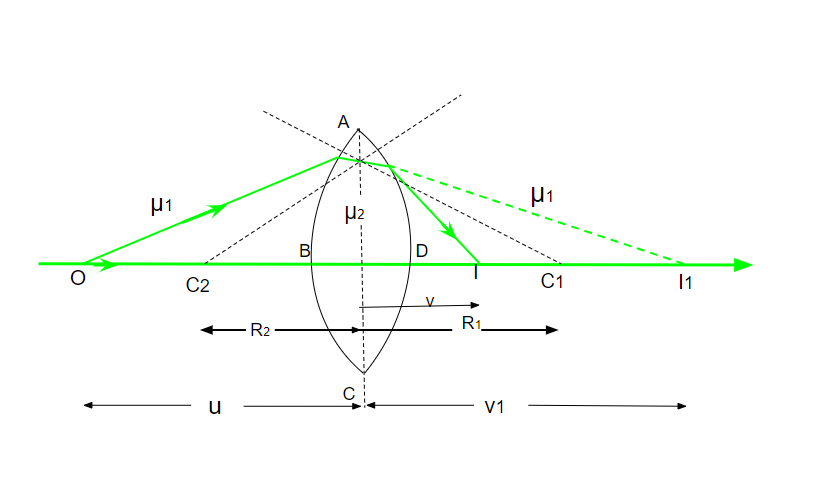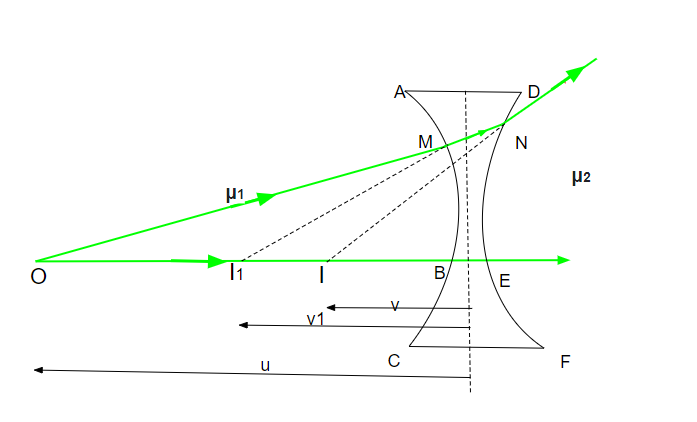# Lens Maker’s Formula

A lens is a piece of polished glass with curved sides for concentrating or dispersing the beam of light rays. A lens is used for magnifying or reducing the image placed in front of it. Scientists use lenses for examining mirror-sized objects. People wear spectacles. The glass used in it is a good example of a lens. There are two types of lenses: concave lens and convex lens. The lens which is thicker at the end than the middle is called the concave lens. The lens which is thicker in the middle than the end is called a convex lens.

Terms to remember

• Pole (p): It is the middle point of the spherical lens or mirror.
• Centre of curvature (C): It is the center of the sphere from which the mirror is formed.
• Principal axis: It is the lines passing through the pole and the center of curvature of the lens.
• Principal focus (F): It is the point at which a narrow beam of light converges or diverges.
• Focal length (f): It is the distance between the focus and the poles of the mirror.

## Lens maker’s formula

Basically, this formula relates to the focal length (f) of a lens to the refractive index of the lens and the radii of the curvature of its two surfaces. The focal length of the lens depends upon the refractive index of the material used for making the lens and the radius of curvature. This formula is used by the manufacturers for making the desired lens; that’s it is called the lens maker’s formula.

Sign conventions:

• The length measured from the optic center to the right-hand side is “positive (+ve)”.
• The length measured from the optic center to the right-hand side is “negative (-ve)”.
• All the distance is measured from the optic center only.

## Lens maker’s formula for convex lens derivationAs shown in the figure above. Consider a convex lens with refractive index μ1 placed in a medium of refractive index μ2. Where μ12. C1 and C2 are the centers of curvature. R1 and R2 are the radii of curvature. v1 is the image distance. u is the object distance. Suppose a point size object O is placed at the principal axis in the medium whose refractive index is μ1. Now suppose the surface ‘ADC’ of the lens is absent, so when ray ‘OM’ is incident on surface ‘ABC’, it refracted along ‘MN’ and the ray ‘MN’ would have met the principal axis at ‘I1‘. So, I1 can be treated as the real image formed by surface ‘ABC’ in the medium of refractive index μ2. So, the relation among μ1, μ2, v1, u, R1 will be,

μ1/v1 – μ1/u =  (μ2 – μ1)/ R1  ⇢ (1)

But when the ray ‘MN’ suffers another refraction at surface ‘ADC’. The emergent ray meets the principal axis at point ‘I’ which is the final image of ‘O’. So, the equation will be,

μ1/v – μ2/v1 = 1 – μ2)/R2 ⇢ (2)

By adding equations (1) and (2),

μ1/v – μ1/u = (μ2 – μ1)[1/R1 – 1/R2]

1/v – 1/u = [(μ2 – μ1)/μ1] [1/R1 – 1/R2] ⇢ (3)

When the object is placed at infinity (u = ∞) then the image will be formed at focus. So, v= f.

1/f =  [(μ2 – μ1)/μ1] [1/R1 – 1/R2] ⇢ (4)  [v = f, u = ∞, 1/∞ = 0]

When lens is placed in air, μ1 = 1, μ2 = u

1/f = (μ1 – 1)[1/R1 -1/R2] ⇢ Final lens maker’s formula

## Lens maker’s formula for concave lensAs shown in the figure above. Consider a thin concave lens of refractive index μ2 is placed in the medium of refractive index μ1. Where μ12. R1 and R2 are the radii of curvature. When a point object ‘O’ is placed at the principal axis in the medium of refractive index μ1. The image formed by the surface ‘ABC will be ‘I1‘. So, the relation among μ1, μ2, v1, u, R1 will be,

μ1/v1 – μ1/u = (μ2 – μ1)/R1 ⇢ (1)

When ray ‘MN’ suffers another refraction at ‘N’. So, ‘I’ is the final virtual image of ‘O’.

μ1/v – μ2/v1 = (μ1 – μ2)/R1 ⇢ (2)

By adding equations (1) and (2),

μ1/v – μ1/u = (μ2 – μ1)[1/R1 – 1/R2]

1/v – 1/u = [(μ2 – μ1)/μ1] [1/R1 – 1/R2]

Suppose the object is placed at infinity; the image formed will be at focus, so (u = ∞) and (v = f),

1/f = [(μ2 – μ1)/μ1] [1/R1 -1/R2]  ⇢ (1/∞ = 0)

When the lens is placed in air μ1 = 1, μ2 = u

1/f = (μ1 – 1)[1/R1 – 1/R2] ⇢  Final lens maker’s formula

## Solved Questions on Lens Maker’s Formula

Question 1: The radius of curvature of each face of the double concave lens made of glass of refractive index 2.5 is 50cm. Calculate the focal length of the lens in the air.

Solution:

μ = 2.5

R1 = -50cm

R2 = +50cm

1/f = (μ – 1)[1/R1 – 1/R2]

1/f = (2.5 – 1) [1/-50 – 1/50]

1/f = 1.5 × (-2/50)

1/f = -3/50

f = -50/3 cm

Therefore, the focus will be -50/3 cm.

Question 2: Find the focal length of the lens whose refractive index is 3, the radius of curvature of each surface is 30cm and -45cm, respectively.

Solution:

μ = 3

R1= 30 cm

R2 = -45cm

By applying lens makers formula,

1/f = (μ – 1) × [1/R1 – 1/R2]

1/f = (3 – 1) × [1/30 – 1/(-45)]

1/f = 2 × (0.033 + 0.022)

1/f = 2×0.055

1/f =  0.111

f = 1/0.111

f = 9.00900901 cm

So, the focal length will be 9.00900901 cm

Question 3: The radii of curvature of the faces of a double convex lens are 20cm and 25cm. Suppose the focal length is 15cm. What is the refractive index of glass?

Solution:

f= 15cm

R1 = +20cm

R2= -25cm

μ =?

using lens maker’s formula

1/f = (μ – 1) [1/R1 – 1/R2]

1/f = (μ-1) [1/20 +1/25]

1/15 = (μ-1) 9/100

(μ-1) = 0.7407

μ= 1.7407

Therefore, refractive index will be 1.7407

Question 4: A biconvex lens has a focal length 3/4 times the radius of curvature of either surface. Calculate the refractive index of the lens.

Solution:

Biconvex lens has same radius of curvature

f= (3/4) R

R1= R

R2 = -R

1/f = (μ – 1) [1/R1 – 1/R2]

(4/3) R = (μ-1) [1/R – 1/R]

(4/3) R = (μ-1) [2/R]

(μ-1) = 4/6

(μ-1) = 0.666

μ = 1.666

Therefore, the refractive index is 1.666

Question 5: Find the radius of curvature of the convex surface of a Plano-convex lens, whose focal length is 0.4m, and the refractive index of the material of the lens is 1.8.

Solution:

μ = 1.8

f = +0.4 m

R1 = ∞

R2 = -R

By applying lens maker’s formula

1/f = (μ – 1) [1/R1 – 1/R2]

1/0.4 = (1.8 -1) [1/∞ + 1/R]

1/0.4 = (0.8) [1/R]

R = (0.4 ×0.8)

R = 0.12 m

Therefore, the radius of curvature 0.12m

Question 6: The radii of curvature of a double convex lens are 25cm and 50cm, and its refractive index is 2.5. Calculate its focal length.

Solution:

μ= 2.5

R1 = +25 cm

R2= -50 cm

1/f = (μ – 1) [1/R1 – 1/R2]

1/f = (2.5 -1) [1/25 +1/50]

1/f = (1.5) [3/50]

f= 50/4.5

f= +11.111cm

Therefore, focal length will be +11.111cm

Question 7: What is the cause of the refraction of light?

As we know, light travels at different speeds in different mediums. The refraction occurs due to the change in speed of light from one medium to another medium.

Question 8: Why do we prefer a magnifying glass of smaller focal length?

As we know that m = 1 + D/f. which means m is inversely proportional to f. So, it clearly suggests that magnifying power will be large when focal length f will be smaller.

Question 9: When does a convex lens behave as a concave lens?

When a lens is placed inside a transparent medium of refractive index greater than that of its own material, then it behaves like a concave lens.

Question 10: What type of lens does an air bubble form inside water?

Air bubble has spherical surface and is surrounded by water of high refractive index. When light passes from water to air it gets diverged. So, air bubbles behave like a concave lens.

Question 11: Under what condition a lens immersed in a transparent liquid is not visible?